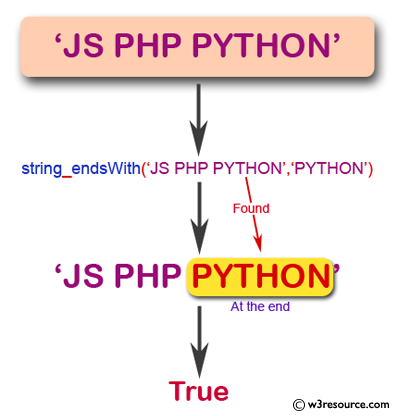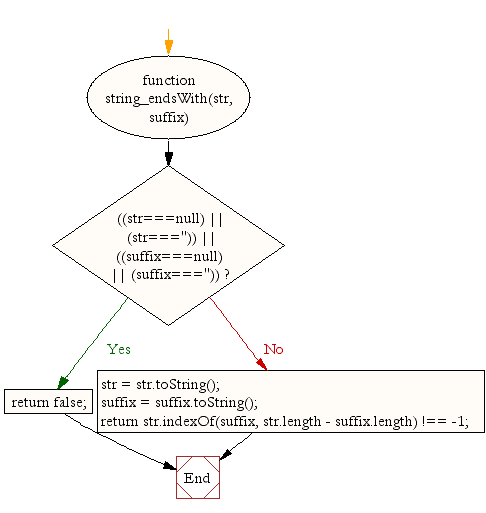# JavaScript: Check if a string ends with specified suffix

## JavaScript String: Exercise-30 with Solution

Write a JavaScript function check if a string ends with specified suffix.

Test Data :
console.log(string_endsWith('JS PHP PYTHON','PYTHON'));
true
console.log(string_endsWith('JS PHP PYTHON',''));
false

Pictorial Presentation:Sample Solution:-

HTML Code:

``````<!DOCTYPE html>
<html>
<meta charset="utf-8">
<title>JavaScript function check if a string ends with specified suffix</title>
<body>

</body>
</html>
```
```

JavaScript Code:

``````function string_endsWith(str, suffix)
{

if (((str===null) || (str==='')) || ((suffix===null) || (suffix==='')))
{
return false;
}
else
{
str = str.toString();
suffix = suffix.toString();
}
return str.indexOf(suffix, str.length -     suffix.length) !== -1;
}

console.log(string_endsWith('JS PHP PYTHON','PYTHON'));

console.log(string_endsWith('JS PHP PYTHON',''));
```
```

Sample Output:

```true
false
```

Flowchart:Live Demo:

See the Pen JavaScript Check if a string ends with specified suffix - string-ex-30 by w3resource (@w3resource) on CodePen.

Improve this sample solution and post your code through Disqus

What is the difficulty level of this exercise?

﻿

## JavaScript: Tips of the Day

JavaScript: Implicit type coercion

```function sum(a, b) {
return a + b;
}

sum(1, '2');
```

JavaScript is a dynamically typed language: we don't specify what types certain variables are. Values can automatically be converted into another type without you knowing, which is called implicit type coercion. Coercion is converting from one type into another.

In this example, JavaScript converts the number 1 into a string, in order for the function to make sense and return a value. During the addition of a numeric type (1) and a string type ('2'), the number is treated as a string. We can concatenate strings like "Hello" + "World", so what's happening here is "1" + "2" which returns "12".

Ref: https://bit.ly/323Y0P6